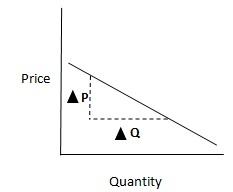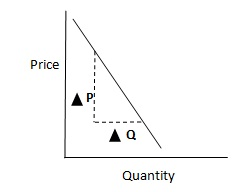# Price elasticity of demand (PED)

Posted in Finance, Accounting and Economics Terms, Total Reads: 4370

## Definition: Price elasticity of demand (PED)

Price elasticity of demand (PED) shows the relationship between the change in the prices and the corresponding change in quantity demanded. It is the ratio of the percentage change in quantity by the percentage change in price.Since price and quantity are negatively correlated for normal goods, this measure is usually negative.This is a case of relatively elastic price demand where absolute PED > 1. The % change in quantity demanded is more than the % change in prices.This is a case of relatively inelastic demand where absolute PED <1. The % change in quantity is less than the % change in price.

A horizontal demand curve would signify completely elastic demand where as a vertical demand curve would signify completely inelastic demand.

Browse the definition and meaning of more terms similar to Price elasticity of demand (PED). The Management Dictionary covers over 7000 business concepts from 6 categories. This definition and concept has been researched & authored by our Business Concepts Team members.

Search & Explore : Management Dictionary

Similar Definitions from same Category: